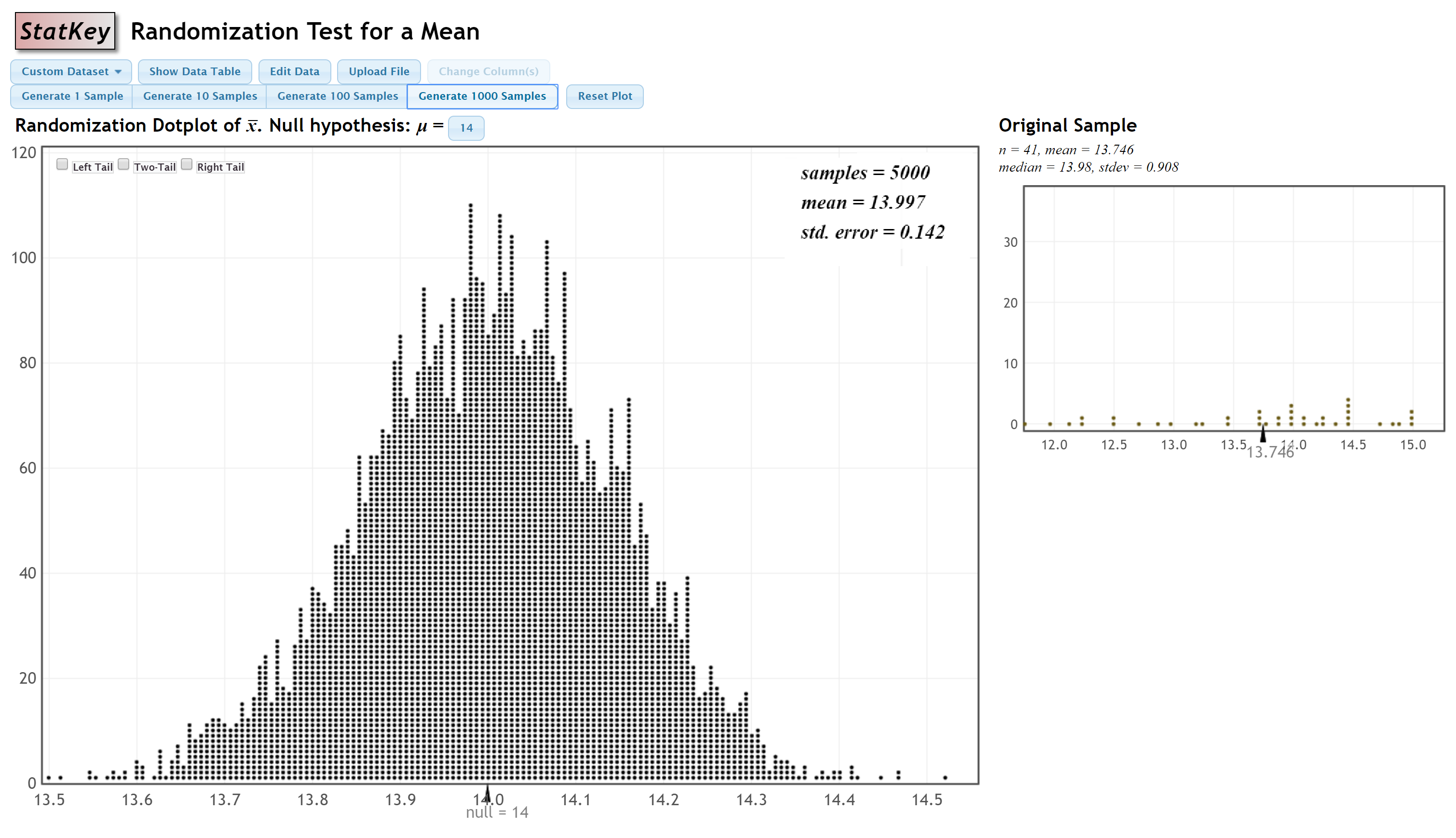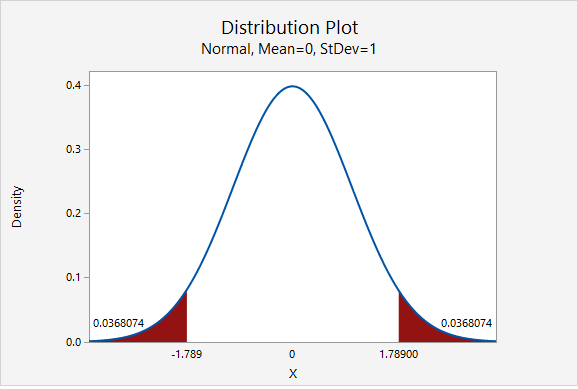# 7.4.1.5 - Example: Mean Quiz Score

Research question: Is the mean quiz score different from 14 in the population?

StatKey was used to construct a randomization distribution:#### Step 1: Check assumptions and write hypotheses

From the given StatKey output, the sampling distribution is approximately normal.

$$H_0\colon \mu = 14$$

$$H_a\colon \mu \ne 14$$

#### Step 2: Calculate the test statistic

$$test\;statistic=\dfrac{sample\;statistic-null\;parameter}{standard\;error}$$

The sample statistic is the mean in the original sample, 13.746 points. The null parameter is 14 points. And, the standard error, 0.142, can be found on the StatKey output.

$$test\;statistic=\dfrac{13.746-14}{0.142}=\dfrac{-0.254}{0.142}=-1.789$$

#### Step 3: Determine the p value

The p value will be the area on the z distribution that is more extreme than the test statistic of -1.789, in the direction of the alternative hypothesis:This was a two-tailed test. The p value is the area in the left and right tails combined: $$p=0.0368074+0.0368074=0.0736148$$

#### Step 4: Make a decision

The p value (0.0736148) is greater than the standard 0.05 alpha level, therefore we fail to reject the null hypothesis.

#### Step 5: State a "real world" conclusion

There is not evidence that the mean quiz score in the population is different from 14 points.